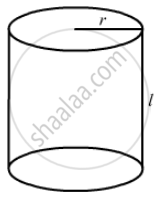Department of Pre-University Education, KarnatakaPUC Karnataka Science Class 11

# The Length and the Radius of a Cylinder Measured with a Slide Callipers Are Found to Be 4.54 Cm and 1.75 Cm Respectively. Calculate the Volume of the Cylinder. - Physics

Sum

The length and the radius of a cylinder measured with a slide callipers are found to be 4.54 cm and 1.75 cm respectively. Calculate the volume of the cylinder.

#### Solution

Length of the cylinder, l = 4.54 cm
Radius of the cylinder, r = 1.75 cm
Volume of the cylinder, V =  pi r^2l=(pi)xx ( 4.54 ) xx ( 1.75 )^2The minimum number of significant digits in a particular term is three. Therefore, the result should have three significant digits, while the other digits should be rounded off.
∴ Volume, V = pi r^2l
= (3.14) × (1.75) × (1.75) × (4.54)
= 43.6577 cm3
Since the volume is to be rounded off to 3 significant digits, we have:
V = 43.7 cm3

Concept: Nature of Physical Laws
Is there an error in this question or solution?

#### APPEARS IN

HC Verma Class 11, Class 12 Concepts of Physics Vol. 1
Chapter 2 Physics and Mathematics
Exercise | Q 33 | Page 30
Share